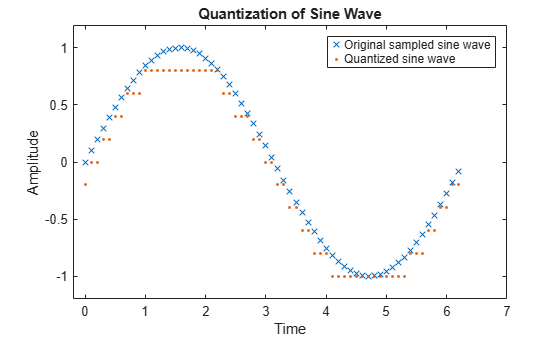# quantiz

Produce quantization index and quantized output value

## Syntax

``index = quantiz(sig,partition)``
``[index,quants] = quantiz(sig,partition,codebook)``
``[index,quants,distor] = quantiz(sig,partition,codebook)``

## Description

````index = quantiz(sig,partition)` returns the quantization levels of input signal `sig` by using the scalar quantization partition specified in input `partition`.```

example

````[index,quants] = quantiz(sig,partition,codebook)` specifies `codebook`, which prescribes a value for each partition in the scalar quantization. `codebook` is a vector whose length must exceed the length of `partition` by one. The function also returns `quants`, which contains the scalar quantization of `sig` and depends on the quantization levels and prescribed values in the codebook.```

example

````[index,quants,distor] = quantiz(sig,partition,codebook)` returns an estimate of the mean square distortion of the quantization data.```

## Examples

collapse all

Generate sample data.

`samp = [-2.4, -1, -0.2, 0, 0.2, 1, 1.2, 2, 2.9, 3, 3.5, 5]`
```samp = 1×12 -2.4000 -1.0000 -0.2000 0 0.2000 1.0000 1.2000 2.0000 2.9000 3.0000 3.5000 5.0000 ```

Create the quantization partition. To specify a partition, list the distinct endpoints of the different ranges of values.

`partition = [0, 1, 3];`

Specify the codebook values.

`codebook = [-1, 0.5, 2, 3]; % Codebook length must be equal to the number of partition intervals`

Perform quantization on the sampled data. Display the quantization index and the corresponding quantized output value of the input data.

`[index,quantized] = quantiz(samp,partition,codebook)`
```index = 1×12 0 0 0 0 1 1 2 2 2 2 3 3 ```
```quantized = 1×12 -1.0000 -1.0000 -1.0000 -1.0000 0.5000 0.5000 2.0000 2.0000 2.0000 2.0000 3.0000 3.0000 ```

Generate a sampled sine wave.

```t = [0:.1:2*pi]; sig = sin(t);```

Create the quantization partition. To specify a partition, list the distinct endpoints of the different ranges of values.

`partition = [-1:.2:1];`

Specify the codebook values.

`codebook = [-1.2:.2:1]; % Codebook length must be equal to the number of partition intervals`

Perform quantization on the sampled sine wave.

`[index,quants] = quantiz(sig,partition,codebook);`

Plot the quantized sine wave and the sampled sine wave.

```plot(t,sig,'x',t,quants,'.') title('Quantization of sine wave') xlabel('Time') ylabel('Amplitude') legend('Original sampled sine wave','Quantized sine wave'); axis([-.2 7 -1.2 1.2])```## Input Arguments

collapse all

Input signal, specified as a vector. This input specifies the sampled signal for this function to perform quantization.

Data Types: `double`

Distinct endpoints of different ranges, specified as a row vector. This input defines several contiguous, nonoverlapping ranges of values within the set of real numbers. The values present in this input must be strictly in ascending order. The length of this vector must be one less than the number of partition intervals.

Example: [`0`, `1`, `3`] partitions the input row vector into the four sets {X: X`0`}, {X: `0` < X`1`}, {X: `1` < X`3`}, and {X: 3 < X}.

Data Types: `double`

Quantization value for each partition, specified as a row vector. This input prescribes a common value for each partition in the scalar quantization. The length of this vector must equal the number of partition intervals, that is, the length of this vector must exceed the length of the `partition` input by one.

Data Types: `double`

## Output Arguments

collapse all

Quantization index of the input signal, returned as a nonnegative row vector. This output determines on which partition interval, each input value is mapped. Each element in `index` is one of the N integers in the range [0, N–1].

If the `partition` input has length N, `index` is a vector whose Kth entry is:

• `0` if `sig`(K) ≤ `partition`(`1`)

• M if `partition`(M) < `sig`(K) ≤ `partition`(M+1)

• N if `partition`(N) ≤ `sig`(`K`)

Output of the quantizer, which contains the quantization values of the input signal, returned as a row vector. The size of `quants` matches that of input argument `sig`. When `codebook` is not specified as an input argument, you can define the codebook values as a vector whose length must exceed the length of the `partition` by one.

`quants` is calculated based on the `codebook` and `index` inputs and is given by `quants`(i) = `codebook`(`index`(i) + 1), where i is an integer between 1 and the length of `sig`.

Mean square distortion of the quantized signal, returned as a positive scalar. You can reduce this distortion by choosing appropriate partition and codebook values. For more information on optimizing partition and codebook values, see the `lloyds` function.

## Version History

Introduced before R2006a